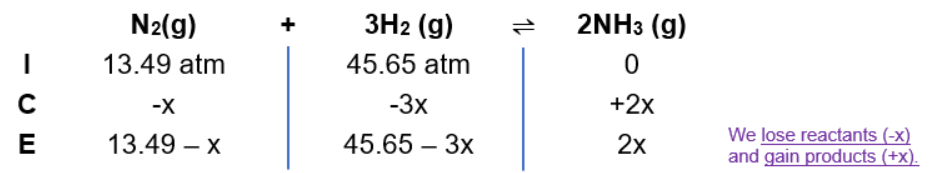# Problem: Ammonia can be synthesized according to the following reaction: N 2(g) + 3H2(g) ⇌ 2NH3(g); Kp = 5.3 x 10–5 at 725 KA 200.0-L reaction container initially contains 1.27 kg of N2 and 0.310 kg of H2 at 725 K. Assuming ideal gas behavior, calculate the mass of NH3 (in g) present in the reaction mixture at equilibrium.

###### FREE Expert Solution

Step 1: Initial Partial Pressure of N2, H2

$\overline{){\mathbf{PV}}{\mathbf{=}}{\mathbf{nRT}}}$

molar mass N2214.00 g/mol = 28.00 g/mol

molar mass H221.01 g/mol = 2.02 g/mol

PN2 = 13.49 atm

PH2 = 45.65 atm

Step 2: ICE ChartStep 3: Kp expression

$\overline{){{\mathbf{K}}}_{{\mathbf{p}}}{\mathbf{=}}\frac{\mathbf{products}}{\mathbf{reactants}}{\mathbf{=}}\frac{{\mathbf{\left[}{\mathbf{NH}}_{\mathbf{3}}\mathbf{\right]}}^{\mathbf{2}}}{\mathbf{\left[}{\mathbf{N}}_{\mathbf{2}}\mathbf{\right]}{\mathbf{\left[}{\mathbf{H}}_{\mathbf{2}}\mathbf{\right]}}^{\mathbf{3}}}}\phantom{\rule{0ex}{0ex}}\phantom{\rule{0ex}{0ex}}{\mathbf{5}}{\mathbf{.}}{\mathbf{3}}{\mathbf{×}}{{\mathbf{10}}}^{\mathbf{-}\mathbf{5}}{\mathbf{=}}\frac{{\left[2x\right]}^{2}}{\left[13.49-x\right]{\left[45.65-3x\right]}^{3}}$

88% (436 ratings)###### Problem Details

Ammonia can be synthesized according to the following reaction: N 2(g) + 3H2(g) ⇌ 2NH3(g); Kp = 5.3 x 10–5 at 725 K

A 200.0-L reaction container initially contains 1.27 kg of N2 and 0.310 kg of H2 at 725 K. Assuming ideal gas behavior, calculate the mass of NH3 (in g) present in the reaction mixture at equilibrium.